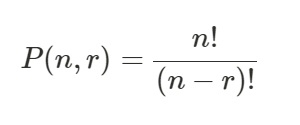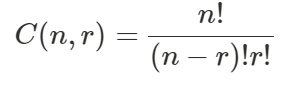# How can SciPy be used to calculate the permutations and combination values in Python?

SciPy can be used to determine the permutation and combination with respect to two values.

A function named ‘perm’ present in the class ‘special’ in ‘SciPy’ is used.

Syntax of ‘perm’ function

scipy.special.perm(N, k)Performing permutation on a set of values has been shown below

## Example

Live Demo

from scipy.special import perm
my_permute = perm(6, 2, exact = True)
print("The permutation of 6 and 2 is ")
print(my_permute)

## Output

The permutation of 6 and 2 is
30

## Explanation

• The required libraries are imported.
• Parameters are passed to the ‘perm’ function that computes the value.
• The value is assigned to a variable.
• This variable is displayed on the console.

## Computing combination of two values in SciPy

A function named ‘comb’ present in the class ‘special’ in ‘SciPy’ is used.

Syntax of ‘comb’ function

scipy.special.comb(N, k)Performing permutation on a set of values has been shown below

## Example

Live Demo

from scipy.special import comb
my_comb = comb(6, 2, exact = True)
print("The combination of 6 and 2 is ")
print(my_comb)

## Output

The combination of 6 and 2 is
15

## Explanation

• The required libraries are imported.
• Parameters are passed to the ‘comb’ function that computes the value.
• The value is assigned to a variable.
• This variable is displayed on the console.